# Independent Complex Samples Z-Test - Comparing Two Means

Where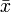$\bar{x}$ and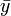$\bar{y}$ are the two means and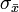$\sigma_\bar{x}$ and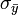$\sigma_\bar{y}$ are their standard errors: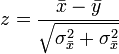$z=\frac{\bar{x}-\bar{y}}{\sqrt{\sigma^2_\bar{x} + \sigma^2_\bar{x}}}$,
where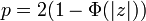$p = 2(1-\Phi(|z|))$ .